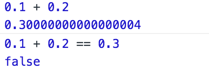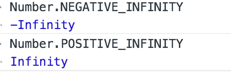# JavaScript中的Number数字类型学习笔记

更新时间：2016年05月26日 17:12:15   作者：Murphywuwu```070//56
079//79
```

```0xA//10
``````-10/0//-Infinity
10/0//Infinity

```isInfinite()判断一个数是否有穷。

```var result = Number.MAX_VALUE + Number.MAX_VALUE
console.log(isFinity(result));//false
```

NaN

```console.log(NaN === NaN)//false

0/0//NaN

```

isNaN:接收一个参数，会尝试将这个值转换为数值，返回布尔值

```console.log(isNaN('10'));//false
console.log(isNaN('bb'));//true
```

Number():使用于任何数值类型。

1.null

```Number(null)//0
undefined
Number(undefined)//NaN
```

2.布尔值

```Number(true)//1
Number(false)//0
```

3.字符串

```Number('bb')//NaN,非数值字符
Number('123')//123,数值字符
Number('')//0,空串
```

4.对象

5.parseInt()/parseFloat():用于将字符串转换为数值。

parseInt():从第一个字符串开始解析，直到解析到非数字字符，可接受两个参数，第二个参数代表不同的进制，默认转换为十进制数。

```parseInt('')//NaN,空串
parseInt(22.4)//22
parseInt('12bu')//12
parseInt('10',2)//2
parseInt('10',8)//8
parseFloat():从第一个字符串开始解析,第一个小数点有效,忽略前导0，只能转换为十进制数值。

parseFloat('')//NaN
parseFloat('090')//90
parseFloat('2.3.4')//2.3

```

• 不区分整数值和浮点数， JavaScript中所有数字均用64位浮点数值标识（IEEE 754）。
• 就像java程序员所熟悉的double类型
• 取值范围在 ±1.7976931348623157 × 10 308 到 ±5 × 10 −324 之间
• 文字:

• 整数
• 十六进制 0x
• 八进制 0
• 浮点表示
• Math类
• 方法
• pow // 求幂
• round //四舍五入
• ceil //向上取整
• floor //向下取整
• abs //求绝对值
• max //最大值
• min //最最小值
• sqrt
• log
• exp
• acos
• asin
• atan
• atan2
• random
• sin
• cos
• 常量
• PI
• E
• LN2
• LN10
• LOG2E
• LOG10E
• PI
• SQRT1_2
• SQRT2
• 无限值
• 正无穷
• Infinity //rw
• Number.POSITIVE_INFINITY // r
• 1/0
• Number.MAX_VALUE + 1
• 负无穷
• Number.NEGATIVE_INFINITY //rw
• -Intifinty //r
• -1/0
• -Number.MAX_VALUE - 1
• NaN
• 不与任何值相等 包括它自己.
• NaN //rw
• Number.NaN //r
• 0/0 //计算结果为NaN
• Zero
• Number.MIN_VALUE/2
• -Number.MIN_VALUE/2
• -1/Infinity
• -0
• Rounding-error

```   var x = .3 - .2
var y = .2 - .1
x == y
x == .1 // => false .3 -.2 不等于 ./
y == .1 // => true .2 - .1 等于 .1

```